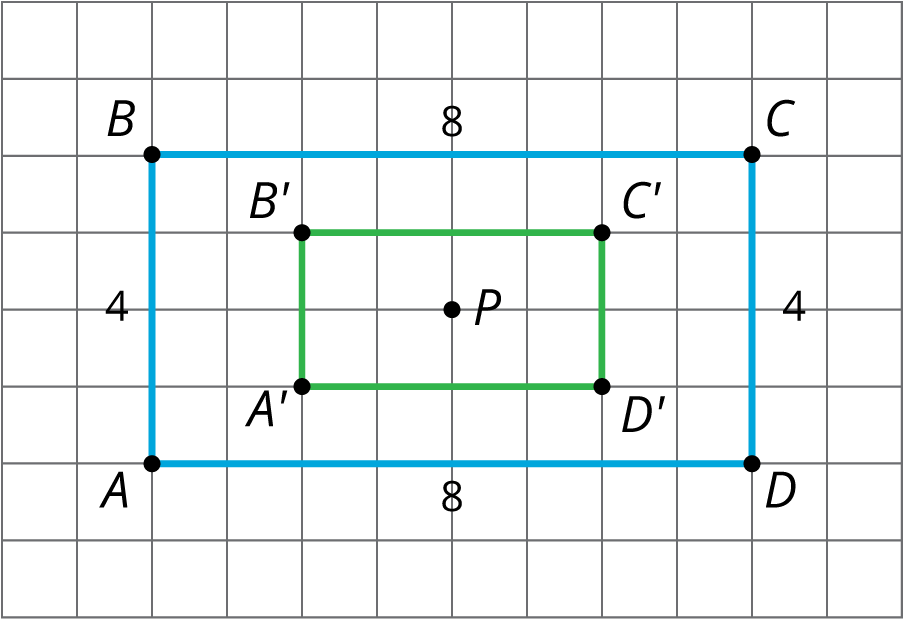# Mathematics Support

Math 8 | Unit 1 2 3 4 5 6 7 8 9

Dilations

Similarity

Slope

## Dilations

This week your student will expand their understanding of transformations to include non-rigid transformations. Specifically, they will learn to make and describe dilations of figures. A dilation is a process to make a scaled copy of a figure, and is described using a center point and a number (the scale factor). The scale factor can be any positive number, including fractions and decimals. If the scale factor is less than 1, the dilated figure is smaller than the original, if it is greater than 1 the dilated figure is larger than the original. In this dilation, the center point P and the scale factor is 12.When dilating figures, the distance from the center of dilation to a point on the figure is multiplied by the scale factor to get the location of the corresponding point. In this example, the distance between center P and Bmultiplied by 12 results in the distance between P and B. Notice also how the side lengths of the dilated figure, ABCD are all exactly 12 the side lengths of the original figure, ABCD, while the angle measures remain the same.

Rectangle A measures 10 cm by 24 cm. Rectangle B is a scaled copy of Rectangle A.

1. If the scale factor is 12, what are the dimensions of Rectangle B?
2. If the scale factor is 3, what are the dimensions of Rectangle B?
3. If Rectangle B has dimensions 15 cm by 36 cm, what is the scale factor?

Solution:

1. Rectangle B has dimensions 5 cm by 12 cm, since 1012=5 and 2412=12.
2. Rectangle B has dimensions 30 cm by 72 cm, since 103=30 and 243=72.
3. The scale factor is 32 since 15÷10=32 and 36÷24=32.

## Similarity

This week your student will investigate what it means for two figures to be similar. Similarity in mathematics means there is a sequence of translations, rotations, reflections, and dilations that takes one figure to the other. When two figures are similar, there are always many different sequences of transformations that can show that they are similar. Here is an example of two similar figures:If we needed to show that these two figures are similar, we can first identify that the scale factor to go from ABDC to EFHG is 34, since 3÷4=4.5÷6=34. Then, using a dilation with scale factor 34, a translation, and a rotation, we can line up an image of ABDC perfectly on top of EFHG.What is the perimeter of quadrilateral EFGH?

Solution:

The perimeter is 42. The scale factor is 1.5, since 9÷6=1.5. This means the side lengths of EFGH are 9, 10.5, 7.5, and 15, which are the values of the corresponding sides of ABCD multiplied by 1.5. We could also just multiply the perimeter of ABCD, 28, by 1.5.

## Slope

This week your student will use what they have learned about similar triangles to define the slope of a line. A slope triangle for a line is a triangle whose longest side lies on the line and whose other two sides are vertical and horizontal. Here are two slope triangles for the line :For lines, it turns out that the quotient of the vertical side length and the horizontal side length of a slope triangle does not depend on the triangle. That is, all slope triangles for a line have the same quotient between their vertical and horizontal side and this number is called the slope of the line. The slope of line  shown here can be written as 68 (from the larger triangle), 34 (from the smaller triangle), 0.75, or any other equivalent value.

By combining what they know about the slope of a line and similar triangles, students will begin writing equations of lines—a skill they will continue to use and refine throughout the rest of the year.

Here is a line with a slope triangle already drawn in.1. What is the slope of the line?
2. Draw another line with a slope of 43 that goes through the point on the left. Include a slope triangle for the new line to show how you know this line has a slope of 43.

Solution:

1. The slope of the line is 37.
2.IM 6–8 Math was originally developed by Open Up Resources and authored by Illustrative Mathematics, and is copyright 2017-2019 by Open Up Resources. It is licensed under the Creative Commons Attribution 4.0 International License (CC BY 4.0). OUR's 6–8 Math Curriculum is available at https://openupresources.org/math-curriculum/.

Disclaimer: This site provides external links and videos as a convenience and for informational purposes only. The appearance of external hyperlinks on the MCPS Family Mathematics Support Center website does not constitute an endorsement by the Montgomery County Public School System of any of the products or opinions contained therein.